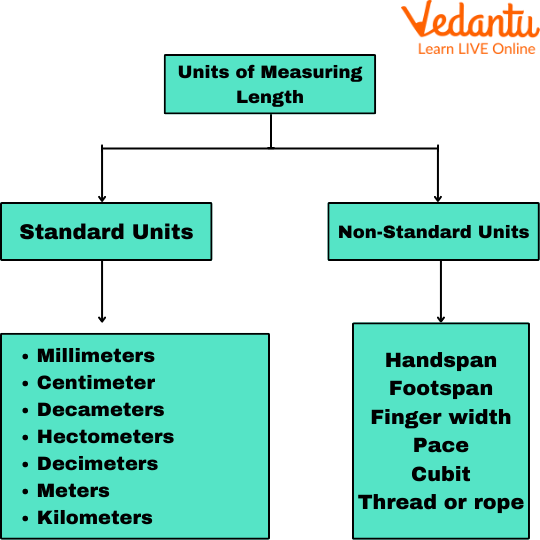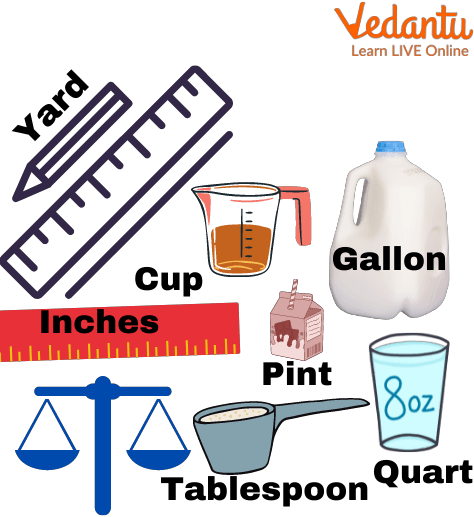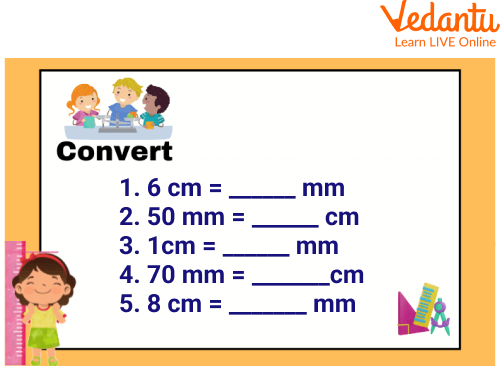Courses
Courses for Kids
Free study material
Free LIVE classes
More

# Measurement Worksheet for 2nd Grade## An Overview of Measurement

Have you ever wondered why we use a certain unit to compare to objects? Like if we want to measure the size of a notebook, we use a scale and measure it in centimeters or inches but we do not use liters or milliliters to measure the length. So what is measurement? It is the comparison of an unknown quantity with a known quantity. The outcome of the measurement is a numeric value with specific units. We can measure the length, mass, capacity(volume), and temperature of any given object using measurement. So in this article, we will learn about measurement, and how we can solve measurement questions for class 2 by solving measuring worksheets for 2nd grade.

## What is Measurement?

Measurement is the act of measuring. It can be understood as a process of defining physical items using numbers. Daily work becomes easier when we know how to measure things, and that is why measurement is a valuable thing to do. Now let's learn more about the units by solving measurement worksheets for 2nd grade.

## Measuring Units

Measuring units are classified into two categories as given below:

A standard unit of measurement is a measurement that enables everyone to understand the object's relationship to the measure. It can be indicated in terms of inches, feet, kilometers, and meters.

• Non-Standard Units of Measurement

In the early days, measurement was done by estimation of body parts, but they weren't accurate because if we use our hand span to measure length, the measurement taken by a different person will vary as our hand spans are not equal. So, hand spans are not standard units of measurement.Units of measuring length

## Measuring Tools

Measuring tools that can be used by kids are given under:

• Thermometers rulers

• Yardsticks

• Scales

• Beakers

• Protractors

• Clocks

• Measuring tape

## Forms of Measurement

Three Forms of Measurement, generally used are given as follows:

• Capacity

The amount that something can hold is defined as its capacity. It typically refers to volume, as in milliliters (ml) or liters (l). A Cup of glass, for example, has a capacity of 500 ml (but is holding only 100 ml). Capacity refers to someone or something's ability to hold something, such as how many pencils can fit in a packet or how much milk a jar can hold.

• Weight (Mass)

The mass of an object is a measure of how much matter it contains. An iron ball, for example, is quite small but has a mass of 1 kg, indicating that it contains a lot of matter. The weight of something is commonly used to determine its mass. However, weight is caused by gravity, whereas your mass remains constant. It is expressed in grams, kilograms, and tonnes.

• Length

The greatest extent of anything in length. It can be measured in terms of meters, centimeters, decametres, kilometers, etc. The length of a pencil is approximately 10 cm, while that of a sharpener is about 3 cm.

We will further learn about these by solving centimeter worksheets for 2nd gradeMeasure units

## Solved Examples

Q 1. Convert 5 kg into grams

Solution: We know that 1 kg =1000 g

As we wish to find more number of kg, so we use multiplication, i.e.

We multiply 1000 g by 5 kg

5 x 1000 g

= 5000 g

Thus 5 kg equals 5000 g.

Q 2. Convert 4l into ml

Solution: We know that 1ltr =1000ml

As we wish to find more numbers of l, so we use multiplication, i.e.

We multiply 1000 ml by 4 l

4 x 1000 ml = 4000 ml

This 4 l equals 4000 ml.

Q 3. Convert 2m into cm

Solution: We know that 1m =100 cm

As we wish to find more numbers of m, so we use multiplication, i.e.

We multiply 100 cm by 2 m

2 x 100 cm = 200 cm

Thus 2 m equals 200 cm.

## Practice Measurement Questions for Class 2

Q 1. Convert the following into milliliters:

1. 7l =_______

Ans: 7000 ml

1. 12l =_______

Ans:12000 ml

Q 2. Convert the following into grams.

(i) 14 kg=________

Ans: 14000 g

(ii) 3 kg =________

Ans:3000 g

Q 1. Mrs Reddy's dogs weigh 5kg. What is the weight of the dog in g?

Ans: 5000 g

Q 2. A doctor prescribed Mr Roy a 500 mg tablet. How much is that in g?

Ans: 0.5 g

Q 3. Kayra’s sharpener has a mass of 20 g. How much is that in mg?

Ans: 20,000 mg## Summary

In this article, we learned about how measurement quantifies the properties of an object or event, allowing us to compare it to other things or events. When dealing with the division of a quantity, the most commonly used word is "measurement". Measuring units along with measurement tools are discussed to clarify the concepts. Some centimeter worksheets for 2nd grade, along with measurement assessment 2nd grade and measurement questions for class 2 are discussed for a better understanding of the concepts.

Last updated date: 20th Sep 2023
Total views: 80.7k
Views today: 0.80k

## FAQs on Measurement Worksheet for 2nd Grade

1. Which of the following is the longest pencil, eraser, or tube light?

As the length of the tube light is maximum, so the longest one is the tube light.

2. What is the use of assigning measuring worksheets for 2nd grade?

Measuring worksheets for 2nd grade helps the students to identify what they have learned and gained through the reading of the above article.

3. Can we measure milk in Kg?

No, milk can't be measured in units of Kg as it is a liquid, so will be measured in terms of liters or milliliters.